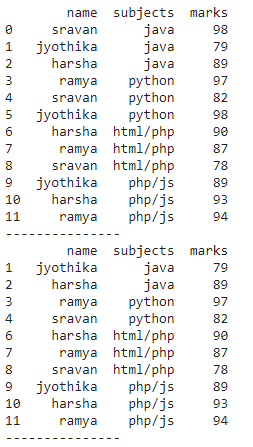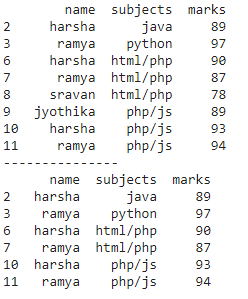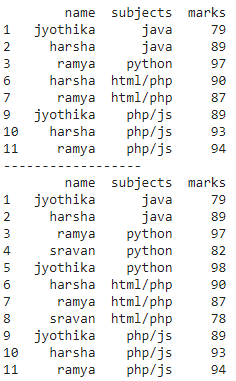How to Drop Rows that Contain a Specific Value in Pandas?

• Last Updated : 22 Nov, 2021

In this article, we will discuss how to drop rows that contain a specific value in Pandas. Dropping rows means removing values from the dataframe we can drop the specific value by using conditional or relational operators.

Method 1: Drop the specific value by using Operators

We can use the column_name function along with the operator to drop the specific value.

Syntax: dataframe[dataframe.column_name operator value]

where

• dataframe is the input dataframe
• column_name is the value of that column to be dropped
• operator is the relational operator
• value is the specific value to be dropped from the particular column

Python3

 # import pandas moduleimport pandas as pd  # create dataframe with 4 columnsdata = pd.DataFrame({      "name": ['sravan', 'jyothika', 'harsha', 'ramya',             'sravan', 'jyothika', 'harsha', 'ramya',             'sravan', 'jyothika', 'harsha', 'ramya'],    "subjects": ['java', 'java', 'java', 'python',                 'python', 'python', 'html/php', 'html/php',                 'html/php', 'php/js', 'php/js', 'php/js'],    "marks": [98, 79, 89, 97, 82, 98, 90,              87, 78, 89, 93, 94]})  # displayprint(data)  print("---------------")  # drop rows where value is 98# by using not equal operatorprint(data[data.marks != 98])  print("---------------")

Output:Method 2: Drop Rows that Contain Values in a List

By using this method we can drop multiple values present in the list, we are using isin() operator. This operator is used to check whether the given value is present in the list or not

Syntax: dataframe[dataframe.column_name.isin(list_of_values) == False]

where

• dataframe is the input dataframe
• column_name is to remove values in this column
• list_of_values is the specific values to be removed

Python3

 # import pandas moduleimport pandas as pd  # create dataframe with 4 columnsdata = pd.DataFrame({      "name": ['sravan', 'jyothika', 'harsha', 'ramya',             'sravan', 'jyothika', 'harsha', 'ramya',              'sravan', 'jyothika', 'harsha', 'ramya'],    "subjects": ['java', 'java', 'java', 'python',                  'python', 'python', 'html/php',                  'html/php', 'html/php', 'php/js',                  'php/js', 'php/js'],    "marks": [98, 79, 89, 97, 82, 98, 90, 87,              78, 89, 93, 94]})  # consider the listlist1 = [98, 82, 79]  # drop rows from above listprint(data[data.marks.isin(list1) == False])  print("---------------")  list2 = ['sravan', 'jyothika']# drop rows from above listprint(data[data.name.isin(list2) == False])

Output:Method 3: Drop rows that contain specific values in multiple columns

We can drop specific values from multiple columns by using relational operators.

Syntax: dataframe[(dataframe.column_name operator value ) relational_operator (dataframe.column_name operator value )]

where

• dataframe is the input dataframe
• column_nam eis the column
• operator is the relational operator

Python3

 # import pandas moduleimport pandas as pd  # create dataframe with 4 columnsdata = pd.DataFrame({      "name": ['sravan', 'jyothika', 'harsha', 'ramya',             'sravan', 'jyothika', 'harsha', 'ramya',             'sravan', 'jyothika', 'harsha', 'ramya'],    "subjects": ['java', 'java', 'java', 'python',                 'python', 'python', 'html/php',                  'html/php', 'html/php', 'php/js',                  'php/js', 'php/js'],    "marks": [98, 79, 89, 97, 82, 98, 90,              87, 78, 89, 93, 94]})  # drop specific values# where marks is 98 and name is sravanprint(data[(data.marks != 98) & (data.name != 'sravan')])  print("------------------")  # drop specific values# where marks is 98 or name is sravanprint(data[(data.marks != 98) | (data.name != 'sravan')])

Output:My Personal Notes arrow_drop_up